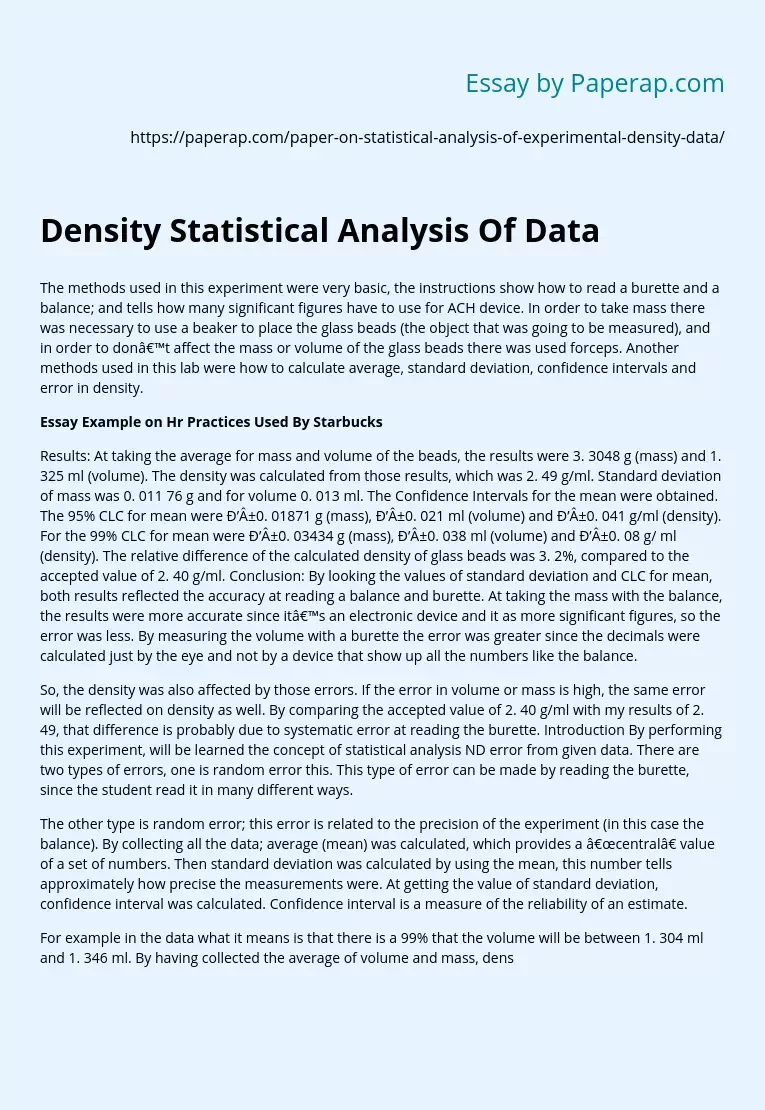# Density Statistical Analysis Of Data

The methods used in this experiment were very basic, the instructions show how to read a burette and a balance; and tells how many significant figures have to use for ACH device. In order to take mass there was necessary to use a beaker to place the glass beads (the object that was going to be measured), and in order to don’t affect the mass or volume of the glass beads there was used forceps. Another methods used in this lab were how to calculate average, standard deviation, confidence intervals and error in density.

## Essay Example on Hr Practices Used By Starbucks

Results: At taking the average for mass and volume of the beads, the results were 3. 3048 g (mass) and 1. 325 ml (volume). The density was calculated from those results, which was 2. 49 g/ml. Standard deviation of mass was 0. 011 76 g and for volume 0. 013 ml. The Confidence Intervals for the mean were obtained. The 95% CLC for mean were В±0. 01871 g (mass), В±0. 021 ml (volume) and В±0.

041 g/ml (density). For the 99% CLC for mean were В±0. 03434 g (mass), В±0. 038 ml (volume) and В±0. 08 g/ ml (density). The relative difference of the calculated density of glass beads was 3. 2%, compared to the accepted value of 2. 40 g/ml. Conclusion: By looking the values of standard deviation and CLC for mean, both results reflected the accuracy at reading a balance and burette. At taking the mass with the balance, the results were more accurate since it’s an electronic device and it as more significant figures, so the error was less. By measuring the volume with a burette the error was greater since the decimals were calculated just by the eye and not by a device that show up all the numbers like the balance.

Get quality help nowWriterBelleVerified

Proficient in: Density4.7 (657)

“ Really polite, and a great writer! Task done as described and better, responded to all my questions promptly too! ”+84 relevant experts are online

So, the density was also affected by those errors. If the error in volume or mass is high, the same error will be reflected on density as well. By comparing the accepted value of 2. 40 g/ml with my results of 2. 49, that difference is probably due to systematic error at reading the burette. Introduction By performing this experiment, will be learned the concept of statistical analysis ND error from given data. There are two types of errors, one is random error this. This type of error can be made by reading the burette, since the student read it in many different ways.

The other type is random error; this error is related to the precision of the experiment (in this case the balance). By collecting all the data; average (mean) was calculated, which provides a “central” value of a set of numbers. Then standard deviation was calculated by using the mean, this number tells approximately how precise the measurements were. At getting the value of standard deviation, confidence interval was calculated. Confidence interval is a measure of the reliability of an estimate.

For example in the data what it means is that there is a 99% that the volume will be between 1. 304 ml and 1. 346 ml. By having collected the average of volume and mass, density was obtained. Density is directly dependent on the results that were obtained from volume and mass. Then from the same data, confidence interval of density was obtained. The last calculation was the percentage difference or error of the experimental value, compared with the accepted value of 2. Ml. At getting this number, it tells how accurate were made the measurements in the experiment.